# 8.3.11 Find the first four nonzero terms in a power series expansion about x = 0... related homework questions

• #### It’s review question, I need this as soon as possible. Thank you 3) For thè diferential equation: (a) The point zo =-1 is an ordinary point. Compute the recursion formula for the coefficients of...It’s review question, I need this as soon as possible. Thank you 3) For thè diferential equation: (a) The point zo =-1 is an ordinary point. Compute the recursion formula for the coefficients of the power series solution centered at zo- -1 and use it to compute the first three nonzero terms of the power series when -1)-s and v(-1)-0....

• #### Dont copié formé thé book oh ya dont copié formé thé book cause you Oiil inde up being triste soi remembré not toi copié frome thé book oh ya

Dont copié formé thé book oh ya dont copié formé thé book cause you Oiil inde up being triste soi remembré not toi copié frome thé book oh ya!translation in english please!

• #### find the first three nonzero terms of the Maclaurin exapnsion kf the function. f(x)=7 sin x Find the first three nonzero terms of the Maclaurin expansion of the function. f(x) = 7 sinx What is the...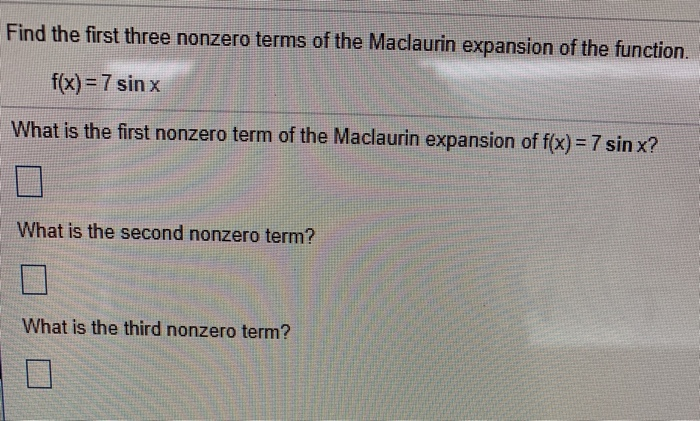find the first three nonzero terms of the Maclaurin exapnsion kf the function. f(x)=7 sin x Find the first three nonzero terms of the Maclaurin expansion of the function. f(x) = 7 sinx What is the first nonzero term of the Maclaurin expansion of f(x) = 7 sin x? 囗 What is the second nonzero term? What is the third...

• #### DSuppose \$39oo is deposited in a savings account that increases exponentially.Detamine thě APv if the acount...DSuppose \$39oo is deposited in a savings account that increases exponentially.Detamine thě APv if the acount increases to \$t020 in 4 years. Ass ume tne interest Vale remains Constant and no additional deposits or Withdrawals are made. (a.) Let pbe the APY. Note tnat if tme inital balaqe is yo, ne year later tne balane is %more. P- 3 (Tpe...

• #### DIFFERENTIAL EQUATIONS: POWER SERIES EXPANSION Find at least the first four non-zero terms in a power series expansion a...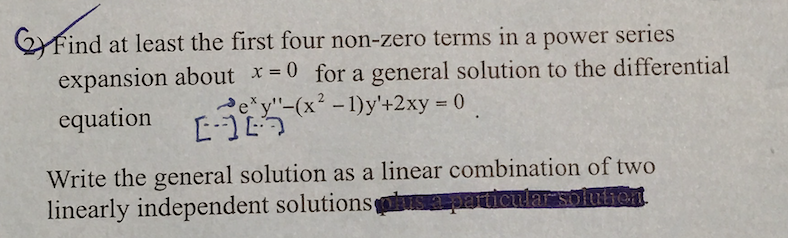DIFFERENTIAL EQUATIONS: POWER SERIES EXPANSION Find at least the first four non-zero terms in a power series expansion about x-0 for a general solution to the differential equation (x2-Dy'+2xy 0 Write the general solution as a linear combination of two linearly independent solutions Find at least the first four non-zero terms in a power series expansion about x-0 for a...

• #### Find the first four nonzero terms in a power series expansion about xo - 2 for the solution to th...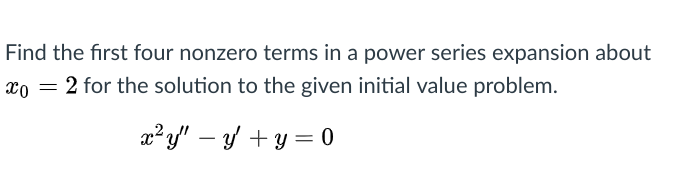Differential equations Find the first four nonzero terms in a power series expansion about xo - 2 for the solution to the given initial value problem Find the first four nonzero terms in a power series expansion about xo - 2 for the solution to the given initial value problem

• #### Find the first four nonzero terms in a power series expansion about x = 0 for...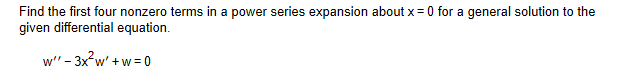Find the first four nonzero terms in a power series expansion about x = 0 for a general solution to the given differential equation. W -3x?w'+w=0

• #### 8.3.14 Find the first four nonzero terms in a power series expansion about x = 0...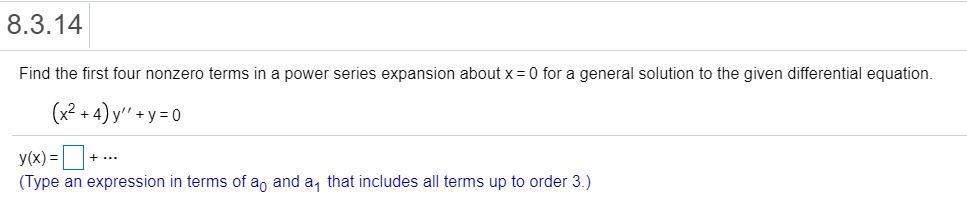8.3.14 Find the first four nonzero terms in a power series expansion about x = 0 for a general solution to the given differential equation. (x2 + 4) y'' +y=0 + ... y(x) = (Type an expression in terms of a, and a that includes all terms up to order 3.)

• #### 8.3.11 Find the first four nonzero terms in a power series expansion about x = 0...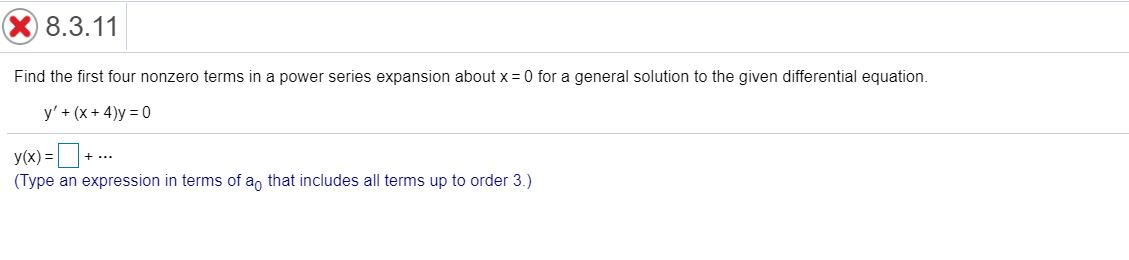8.3.11 Find the first four nonzero terms in a power series expansion about x = 0 for a general solution to the given differential equation. y' + (x + 4)y=0 +... y(x)=+. (Type an expression in terms of a, that includes all terms up to order 3.)

• #### X 8.4.11 Question Help Find the first four nonzero terms in a power series expansion about...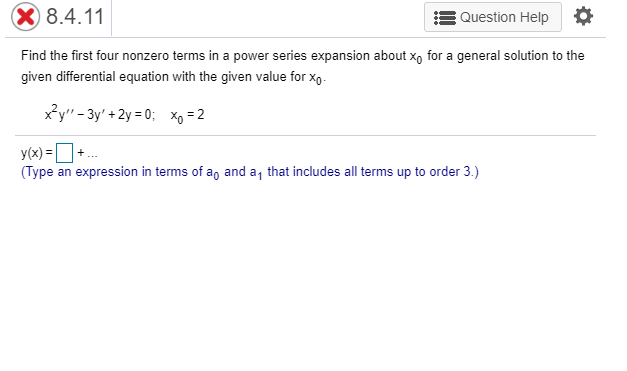X 8.4.11 Question Help Find the first four nonzero terms in a power series expansion about xo for a general solution to the given differential equation with the given value for Xo- x?y" - 3y' +2y = 0; Xo = 2 y(x)=+ (Type an expression in terms of a, and a that includes all terms up to order 3.)

• #### X 8.4.14 Question Help Find the first four nonzero terms in a power series expansion of...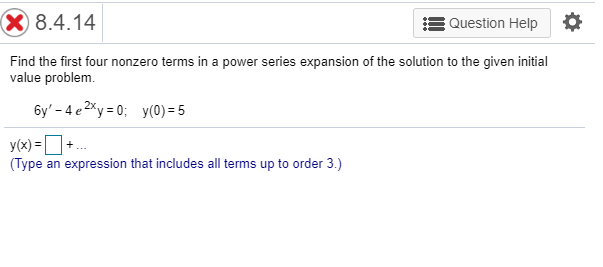X 8.4.14 Question Help Find the first four nonzero terms in a power series expansion of the solution to the given initial value problem 6y' - 4 e 2xy = 0; y(0)=5 y(x) = (Type an expression that includes all terms up to order 3.)

• #### 8.4.9 Question Help Find the first four nonzero terms in a power series expansion about xo...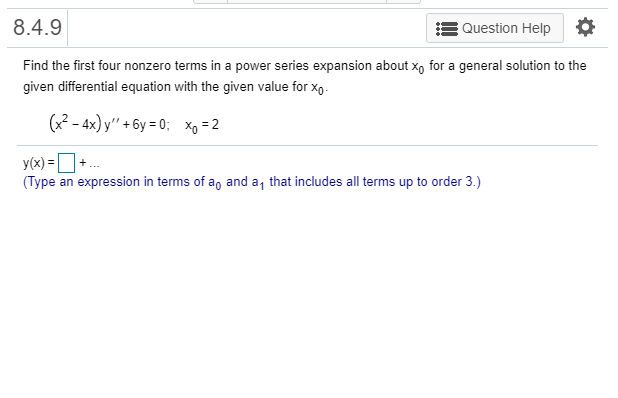8.4.9 Question Help Find the first four nonzero terms in a power series expansion about xo for a general solution to the given differential equation with the given value for Xo- (x2 - 4x) y" + 6y = 0; xp = 2 + y(x)= (Type an expression in terms of a, and a that includes all terms up to order...

• #### Find the first four nonzero terms in a power series expansion about xo for a general...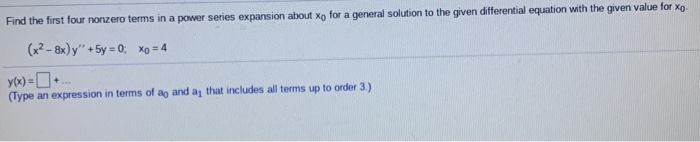Find the first four nonzero terms in a power series expansion about xo for a general solution to the given differential equation with the given value for Xo. (x2 - 8x y + 5y = 0, xo = 4 (Type an expression in terms of ag and a, that includes all terms up to order 3.)

• #### 1. Find the first three nonzero terms in a power series expansion about Xo = 0...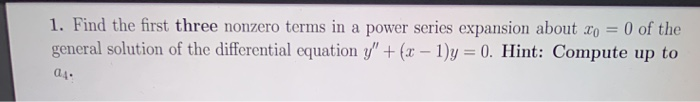1. Find the first three nonzero terms in a power series expansion about Xo = 0 of the general solution of the differential equation y" + (x - 1)y = 0. Hint: Compute up to 04.

• #### 2. Find the first three nonzero terms in a power series expansion about to = 0...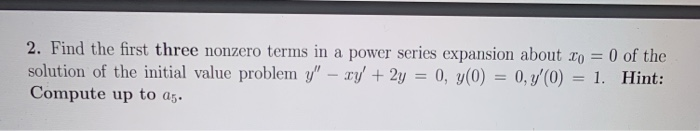2. Find the first three nonzero terms in a power series expansion about to = 0 of the solution of the initial value problem y" - xy + 2y = 0, y(0) = 0,7'0) = 1. Hint: Compute up to 25.

• #### 1. Find the first three nonzero terms in a power series expansion about Xo = 0...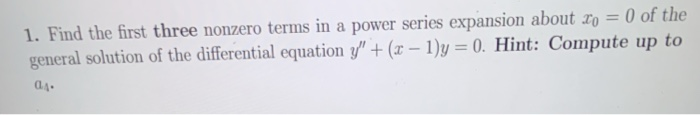1. Find the first three nonzero terms in a power series expansion about Xo = 0 of the general solution of the differential equation y" + (x - 1)y = 0. Hint: Compute up to 01.

• #### 4. Find the first three nonzero terms in a power series expansion about xo = 0...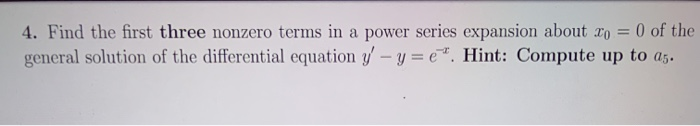4. Find the first three nonzero terms in a power series expansion about xo = 0 of the general solution of the differential equation y' - y=e". Hint: Compute up to 25.

• #### 3. Find the first three nonzero terms in a power series expansion about to 1 of...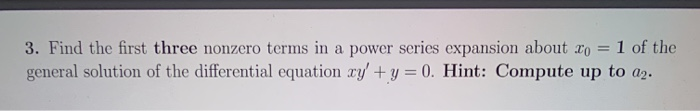3. Find the first three nonzero terms in a power series expansion about to 1 of the general solution of the differential equation xy + y = 0. Hint: Compute up to a2.

• #### Nts) Use the method of Frobenius to find the first four nonzero terms in the series expansion abo...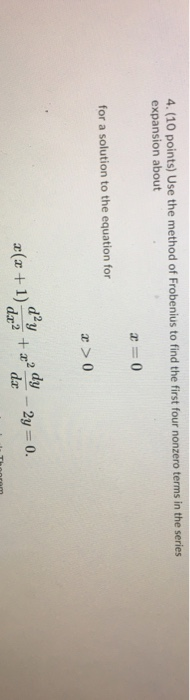nts) Use the method of Frobenius to find the first four nonzero terms in the series expansion about for a solution to the equation for r >0 1 dz2 nts) Use the method of Frobenius to find the first four nonzero terms in the series expansion about for a solution to the equation for r >0 1 dz2

• #### Problem 8. (7 points)Find the first four nonzero terms of the power series solution in powers...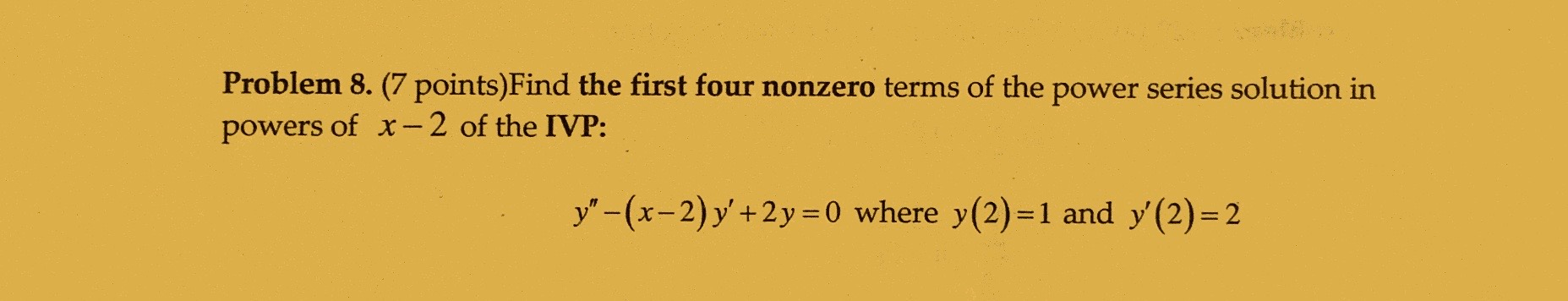Problem 8. (7 points)Find the first four nonzero terms of the power series solution in powers of x-2 of the IVP: y"-(x-2) y'+2y=0 where y(2)=1 and y'(2)= 2

Free Homework App